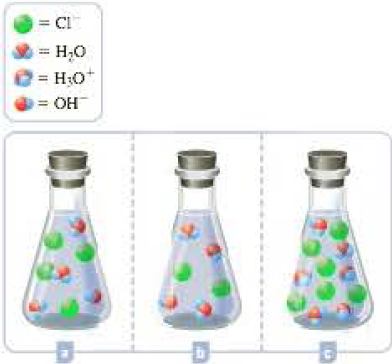Chapter 16, Problem 16.32QPGeneral Chemistry - Standalone boo...

11th Edition
Steven D. Gammon + 7 others
ISBN: 9781305580343

Solutions

Chapter
SectionGeneral Chemistry - Standalone boo...

11th Edition
Steven D. Gammon + 7 others
ISBN: 9781305580343
Textbook Problem

The three flasks shown below depict the titration of an aqueous NaOH solution with HCl at different points. One represents the titration prior to the equivalence point, another represents the titration at the equivalence point, and the other represents the titration past the equivalence point. (Sodium ions and solvent water molecules have been omitted for clarity.) a Write the balanced chemical equation for the titration. b Label each of the beakers shown to indicate which point in the titration they represent. c For each solution, indicate whether you expect it to be acidic, basic, or neutral.(a)

Interpretation Introduction

Interpretation:

For the titration of aqueous NaOH solution with HCl ,

The balanced chemical equation for the titration has to be written.

Concept Introduction:

Equivalence point:

The equivalence point in titration is the point where the amount of standard titrant solution (in moles) and the unknown concentration analyte solution (in moles) becomes equal.

In other words, the equivalence point is the point obtained in a titration once a stoichiometric amount of reactant has been added.

Explanation

To Write: The balanced equation for the given titration

Given data:

The three flasks shown below Figure 1, depict the titration  of an aqueous NaOH solution with HCl at different points.

Figure 1

One beaker represents the titration before the equivalence point

One more represents the titration at the equivalence point

Another one represents the titration past the equivalence point

(b)

Interpretation Introduction

Interpretation:

For the titration of aqueous NaOH solution with HCl ,

The point of titration represented in each beaker has to be labeled

Concept Introduction:

Equivalence point:

The equivalence point in titration is the point where the amount of standard titrant solution (in moles) and the unknown concentration analyte solution (in moles) becomes equal.

In other words, the equivalence point is the point obtained in a titration once a stoichiometric amount of reactant has been added.

(c)

Interpretation Introduction

Interpretation:

For the titration of aqueous NaOH solution with HCl ,

Whether each solutions in the beaker represent acidic, basic or neutral has to be indicated.

Concept Introduction:

Equivalence point:

The equivalence point in titration is the point where the amount of standard titrant solution (in moles) and the unknown concentration analyte solution (in moles) becomes equal.

In other words, the equivalence point is the point obtained in a titration once a stoichiometric amount of reactant has been added.

Still sussing out bartleby?

Check out a sample textbook solution.

See a sample solution

The Solution to Your Study Problems

Bartleby provides explanations to thousands of textbook problems written by our experts, many with advanced degrees!

Get Started

The smallest unit of any substance is the _____. a. atom b. molecule c. cell

Biology: The Unity and Diversity of Life (MindTap Course List)

Write the molecular formula for each alkane.

Introduction to General, Organic and Biochemistry

are removed from new mRNAs. a. Introns b. Exons c. Telomeres d. Amino adds

Biology: The Unity and Diversity of Life (MindTap Course List)